# Relational Algebra Chapter 07 Relational Algebra Calculus Relational

• Slides: 54
Download presentation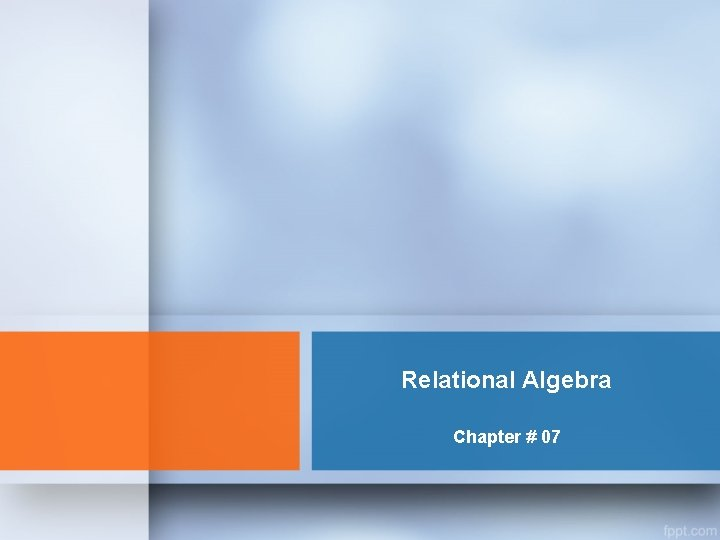Relational Algebra Chapter # 07Relational Algebra & Calculus • Relational algebra and relational calculus are formal languages associated with the relational model. • Informally, relational algebra is a (high-level) procedural language and relational calculus a non-procedural language. – Relational Algebra: More operational (procedural), very useful for representing execution plans. – Relational Calculus: Lets users describe what they want, rather than how to compute it: Non-operational, declarative. • However, formally both are equivalent to one another.Relational Algebra • The basic set of operations for the relational model is the relational algebra. – enable the specification of basic retrievals • Relational algebra operations work on one or more relations to define another relation without changing the original relations. • Both operands and results are relations, so output from one operation can become input to another operation. • Allows expressions to be nested, just as in arithmetic.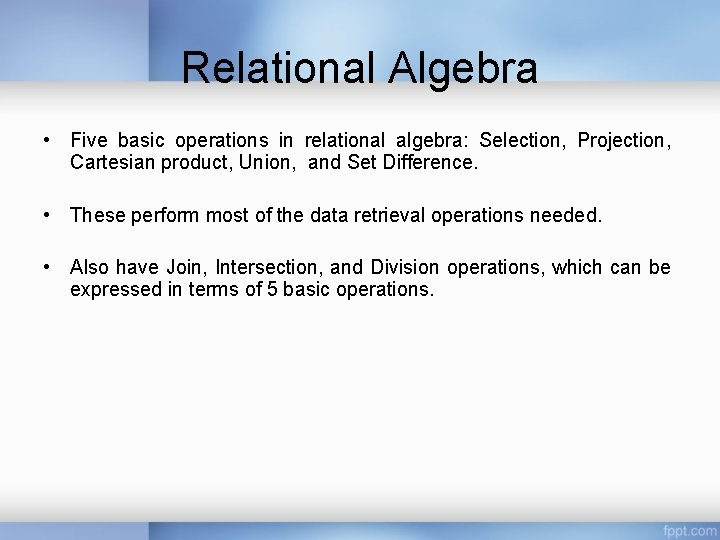Relational Algebra • Five basic operations in relational algebra: Selection, Projection, Cartesian product, Union, and Set Difference. • These perform most of the data retrieval operations needed. • Also have Join, Intersection, and Division operations, which can be expressed in terms of 5 basic operations.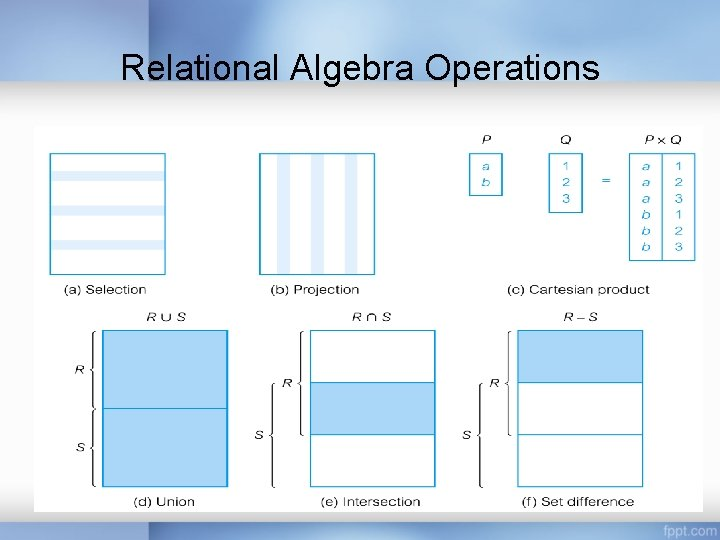Relational Algebra Operations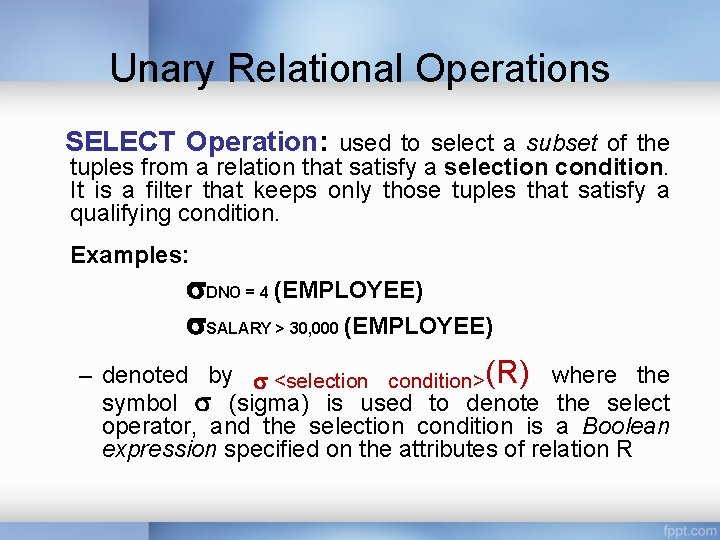Unary Relational Operations SELECT Operation: used to select a subset of the tuples from a relation that satisfy a selection condition. It is a filter that keeps only those tuples that satisfy a qualifying condition. Examples: DNO = 4 (EMPLOYEE) SALARY > 30, 000 (EMPLOYEE) – denoted by <selection condition>(R) where the symbol (sigma) is used to denote the select operator, and the selection condition is a Boolean expression specified on the attributes of relation R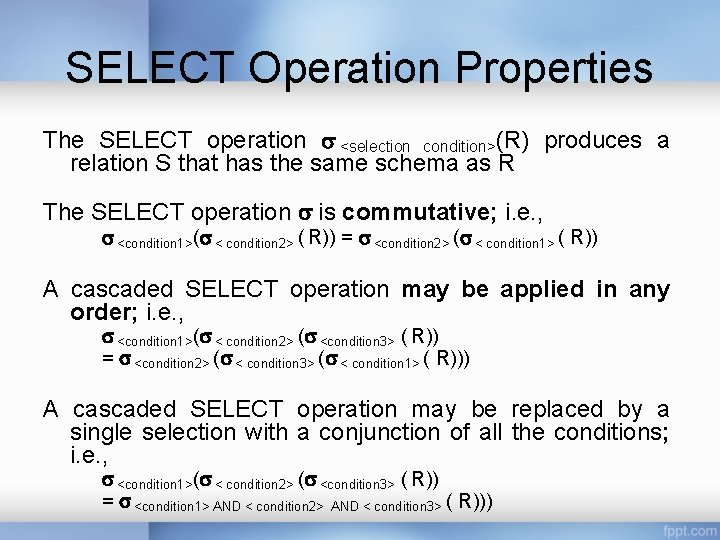SELECT Operation Properties The SELECT operation <selection condition>(R) produces a relation S that has the same schema as R The SELECT operation is commutative; i. e. , <condition 1>( < condition 2> ( R)) = <condition 2> ( < condition 1> ( R)) A cascaded SELECT operation may be applied in any order; i. e. , <condition 1>( < condition 2> ( <condition 3> ( R)) = <condition 2> ( < condition 3> ( < condition 1> ( R))) A cascaded SELECT operation may be replaced by a single selection with a conjunction of all the conditions; i. e. , <condition 1>( < condition 2> ( <condition 3> ( R)) = <condition 1> AND < condition 2> AND < condition 3> ( R)))Selection Condition Operators: <, , , >, =, Simple selection condition: – – <attribute> operator <constant> <attribute> operator <attribute> <condition> AND <condition> OR <condition> – NOT <condition>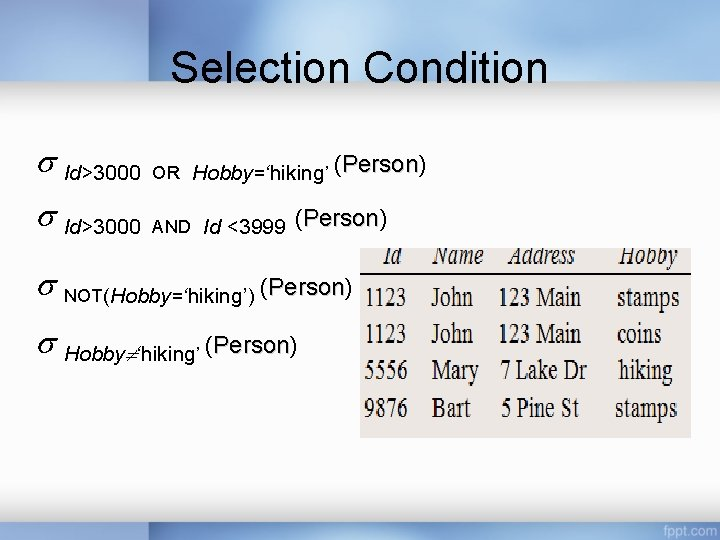Selection Condition Id>3000 Person OR Hobby=‘hiking’ (Person) Id>3000 AND Id <3999 (Person) Person NOT(Hobby=‘hiking’) (Person) Person Hobby ‘hiking’ (Person) Person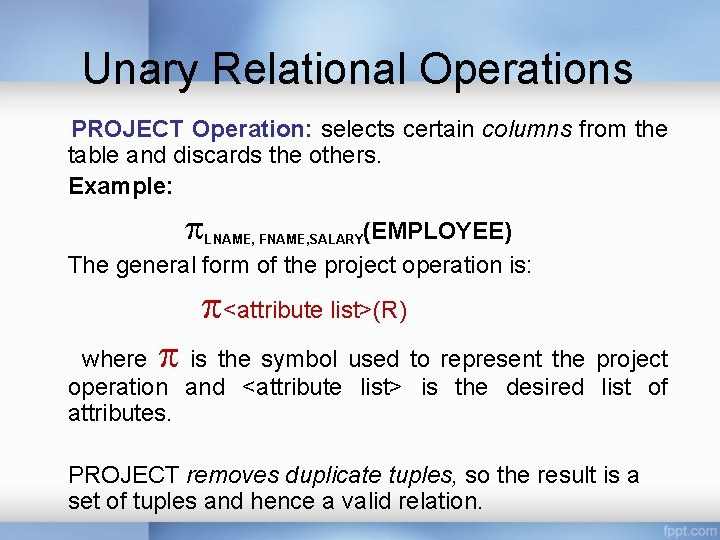Unary Relational Operations PROJECT Operation: selects certain columns from the table and discards the others. Example: (EMPLOYEE) The general form of the project operation is: LNAME, FNAME, SALARY <attribute list>(R) where is the symbol used to represent the project operation and <attribute list> is the desired list of attributes. PROJECT removes duplicate tuples, so the result is a set of tuples and hence a valid relation.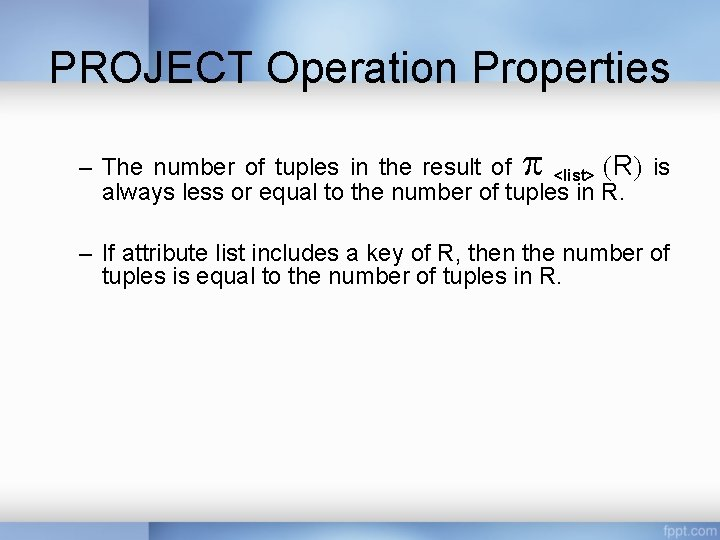PROJECT Operation Properties – The number of tuples in the result of <list> R is always less or equal to the number of tuples in R. – If attribute list includes a key of R, then the number of tuples is equal to the number of tuples in R.SELECT and PROJECT Operations (a) s(DNO=4 AND SALARY>25000) OR (DNO=5 AND SALARY>30000)(EMPLOYEE) (b) LNAME, FNAME, SALARY(EMPLOYEE)RENAME operation Rename attributes in intermediate results ρS(B 1, B 2, . . . , Bn)(R) ρS(R) ρ(B 1, B 2, . . . , Bn)(R) where the symbol ρ(rho) is used to denote the RENAME operator, S is the new relation name, and B 1, B 2, . . . , Bn are the new attribute names.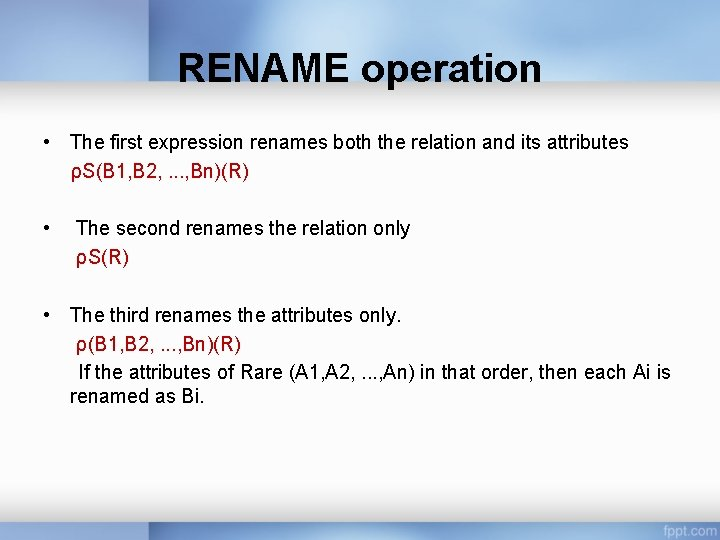RENAME operation • The first expression renames both the relation and its attributes ρS(B 1, B 2, . . . , Bn)(R) • The second renames the relation only ρS(R) • The third renames the attributes only. ρ(B 1, B 2, . . . , Bn)(R) If the attributes of Rare (A 1, A 2, . . . , An) in that order, then each Ai is renamed as Bi.Relational Algebra Operations from Set Theory • The UNION, INTERSECTION, and MINUS Operations • The CARTESIAN PRODUCT (or CROSS PRODUCT) Operation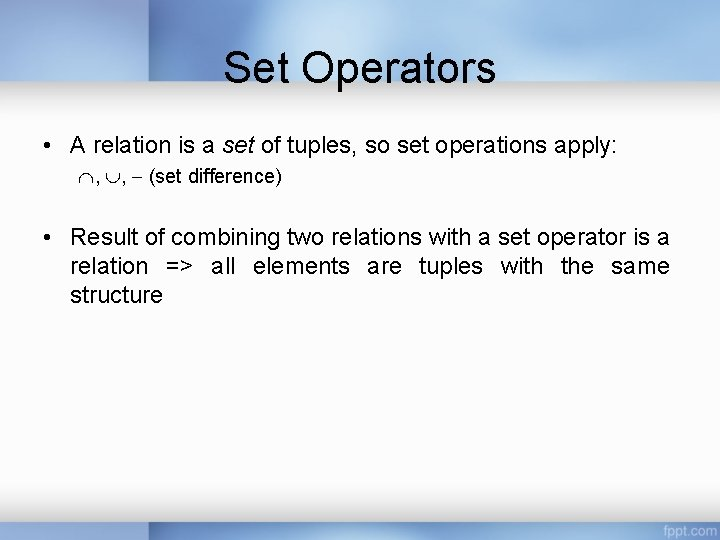Set Operators • A relation is a set of tuples, so set operations apply: , , (set difference) • Result of combining two relations with a set operator is a relation => all elements are tuples with the same structure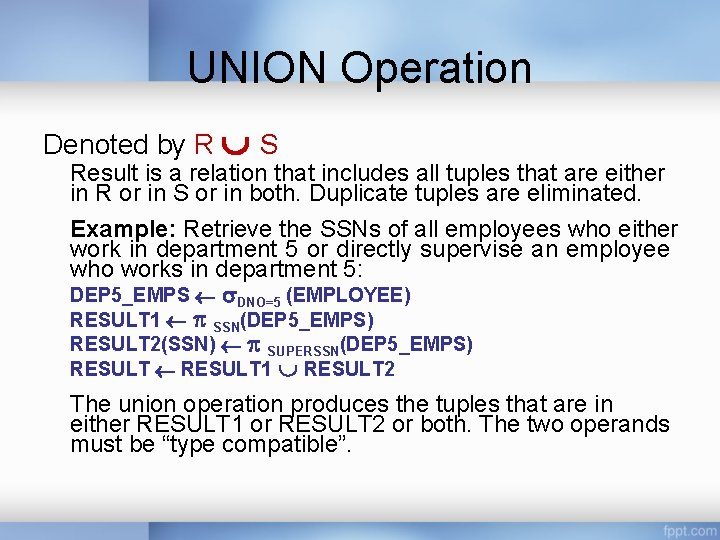UNION Operation Denoted by R S Result is a relation that includes all tuples that are either in R or in S or in both. Duplicate tuples are eliminated. Example: Retrieve the SSNs of all employees who either work in department 5 or directly supervise an employee who works in department 5: DEP 5_EMPS DNO=5 (EMPLOYEE) RESULT 1 SSN(DEP 5_EMPS) RESULT 2(SSN) SUPERSSN(DEP 5_EMPS) RESULT 1 RESULT 2 The union operation produces the tuples that are in either RESULT 1 or RESULT 2 or both. The two operands must be “type compatible”.UNION Operation Type (Union) Compatibility The operand relations R 1(A 1, A 2, . . . , An) and R 2(B 1, B 2, . . . , Bn) must have the same number of attributes, and the domains of corresponding attributes must be compatible, i. e. – dom(Ai) = dom(Bi) for i=1, 2, . . . , n.Intersection Operation Denoted by R ∩ S The intersection operation also has the requirement that both the relations should be union compatible, which means they are of same degree and same domains. • If R and S are two relations and we take intersection of these two relations then the resulting relation would be the set of tuples, which are in both R and S. Just like union intersection is also commutative. • R ∩ S = S ∩R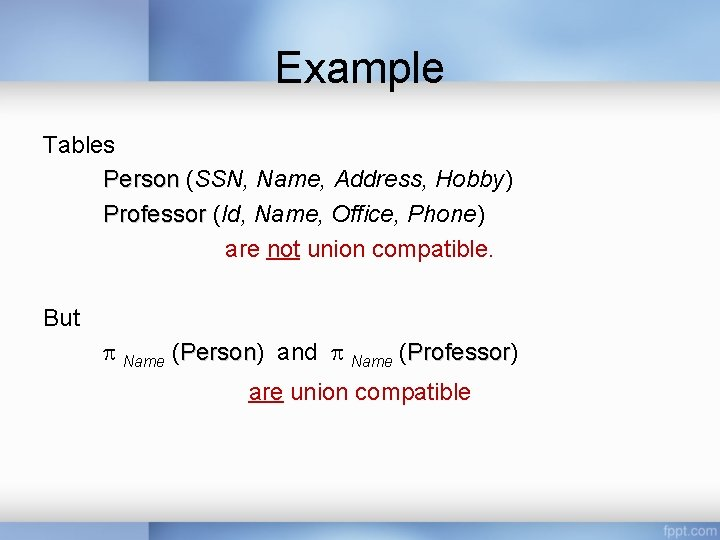Example Tables Person (SSN, Name, Address, Hobby) Professor (Id, Name, Office, Phone) are not union compatible. But Name (Person) Person and Name (Professor) Professor are union compatibleSet Difference (or MINUS) Operation The result of this operation, denoted by R - S, is a relation that includes all tuples that are in R but not in S. The two operands must be "type compatible”.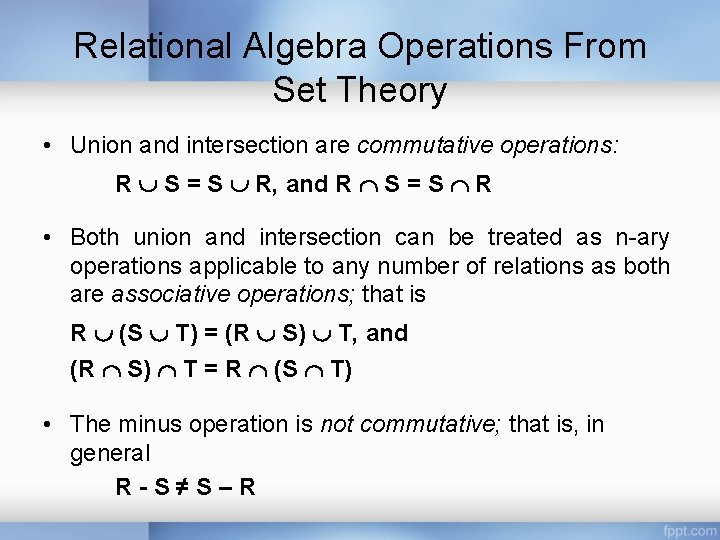Relational Algebra Operations From Set Theory • Union and intersection are commutative operations: R S = S R, and R S = S R • Both union and intersection can be treated as n-ary operations applicable to any number of relations as both are associative operations; that is R (S T) = (R S) T, and (R S) T = R (S T) • The minus operation is not commutative; that is, in general R-S≠S–RRelational Algebra Operations from Set TheoryCartesian (Cross) Product • If R and S are two relations, R S is the set of all concatenated tuples <x, y>, where x is a tuple in R and y is a tuple in S – R and S need not be union compatible • R S is expensive to compute A B x 1 x 2 x 3 x 4 C D y 1 y 2 y 3 y 4 R S A B C D x 1 x 2 y 1 y 2 x 1 x 2 y 3 y 4 x 3 x 4 y 1 y 2 x 3 x 4 y 3 y 4 R SBinary Relational Operations: JOIN and DIVISION • The JOIN Operation • The EQUIJOIN and NATURAL JOIN variations of JOIN • The DIVISION Operation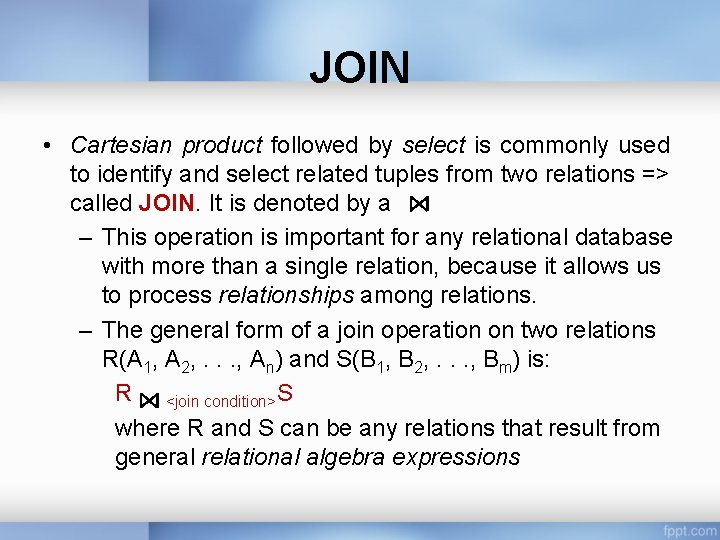JOIN • Cartesian product followed by select is commonly used to identify and select related tuples from two relations => called JOIN. It is denoted by a – This operation is important for any relational database with more than a single relation, because it allows us to process relationships among relations. – The general form of a join operation on two relations R(A 1, A 2, . . . , An) and S(B 1, B 2, . . . , Bm) is: R <join condition>S where R and S can be any relations that result from general relational algebra expressions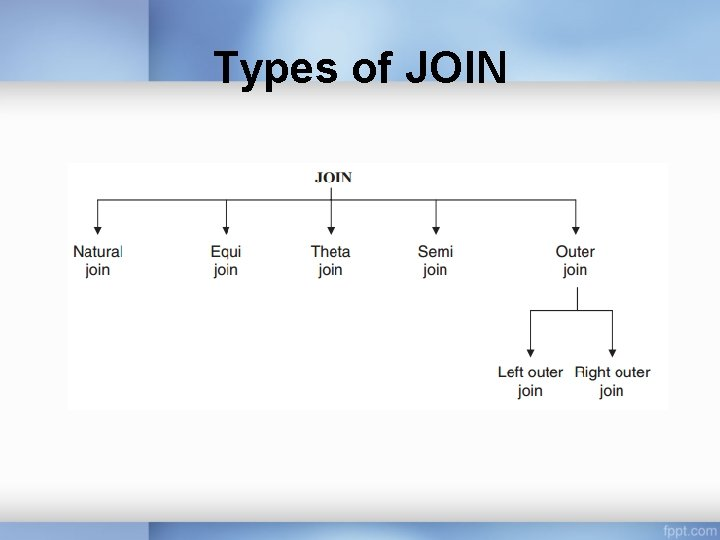Types of JOIN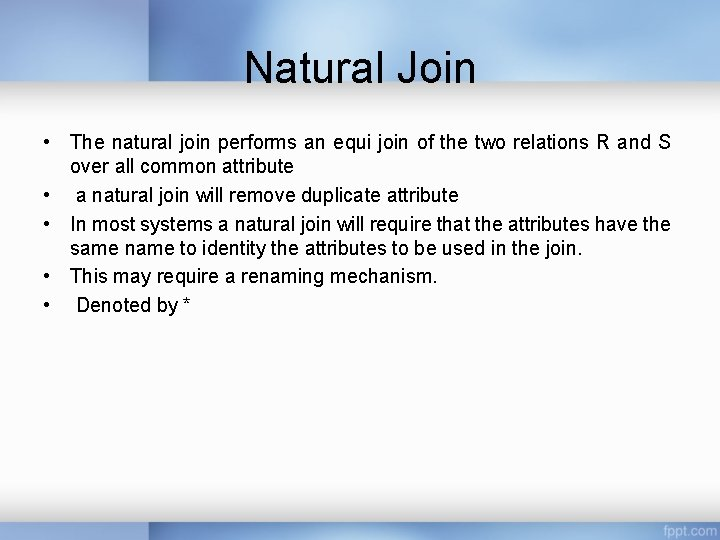Natural Join • The natural join performs an equi join of the two relations R and S over all common attribute • a natural join will remove duplicate attribute • In most systems a natural join will require that the attributes have the same name to identity the attributes to be used in the join. • This may require a renaming mechanism. • Denoted by *Natural Join • Input: Two relations (tables) R and S • Notation: R S • Purpose: Relate rows from second table and – Enforce equality on all column attributes – Eliminate one copy of common attributeNATURAL JOINNATURAL JOIN In the following example, first we rename the Dnumber attribute Of DEPARTMENT to Dnum—so that it has the same name as the Dnum attribute in PROJECT—and then we apply NATURAL JOIN: PROJ_DEPT ← PROJECT *ρ(Dname, Dnum, Mgr_ssn, Mgr_start_date)(DEPARTMENT)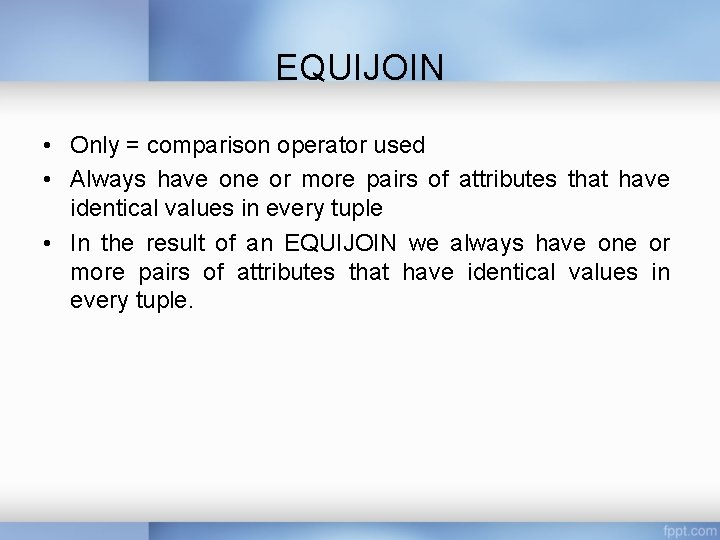EQUIJOIN • Only = comparison operator used • Always have one or more pairs of attributes that have identical values in every tuple • In the result of an EQUIJOIN we always have one or more pairs of attributes that have identical values in every tuple.EQUIJOIN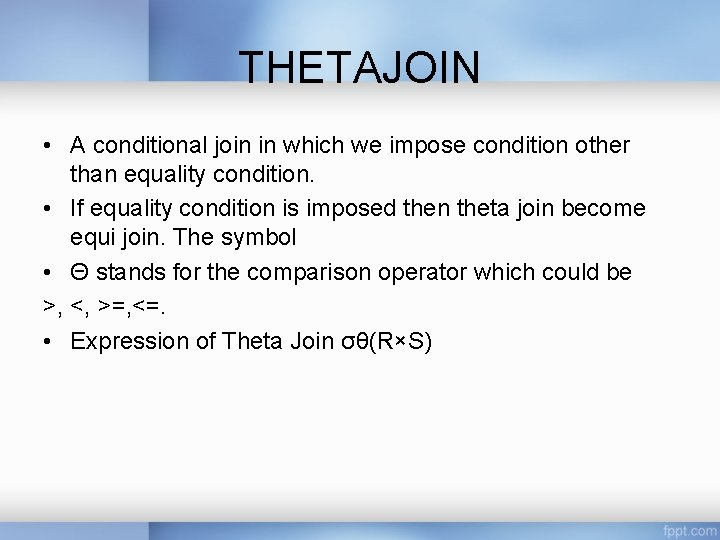THETAJOIN • A conditional join in which we impose condition other than equality condition. • If equality condition is imposed then theta join become equi join. The symbol • Θ stands for the comparison operator which could be >, <, >=, <=. • Expression of Theta Join σθ(R×S)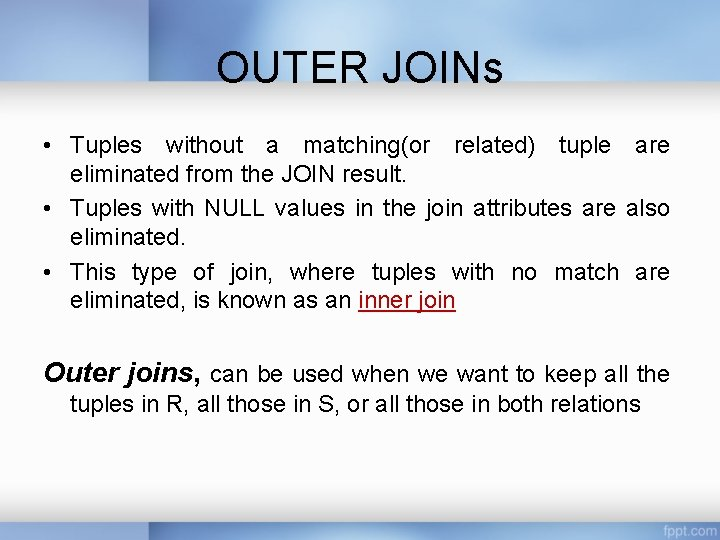OUTER JOINs • Tuples without a matching(or related) tuple are eliminated from the JOIN result. • Tuples with NULL values in the join attributes are also eliminated. • This type of join, where tuples with no match are eliminated, is known as an inner join Outer joins, can be used when we want to keep all the tuples in R, all those in S, or all those in both relationsOUTER JOINs Types • LEFT OUTER JOIN • RIGHT OUTER JOIN • FULL OUTER JOINOUTER JOINs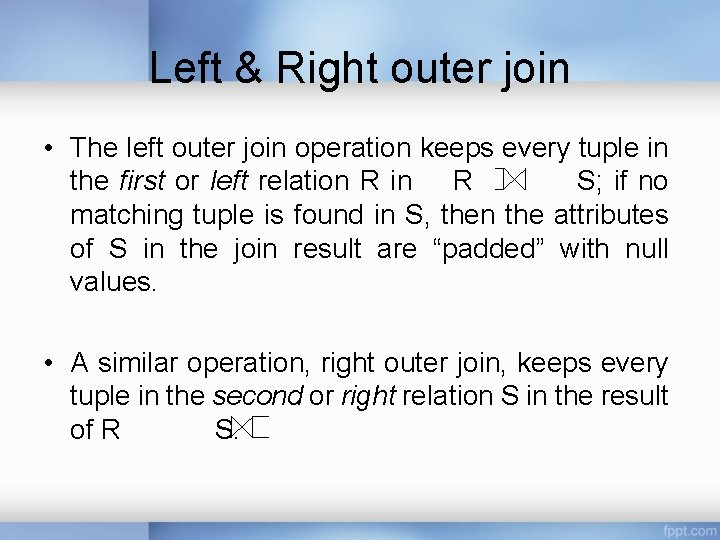Left & Right outer join • The left outer join operation keeps every tuple in the first or left relation R in R S; if no matching tuple is found in S, then the attributes of S in the join result are “padded” with null values. • A similar operation, right outer join, keeps every tuple in the second or right relation S in the result of R S.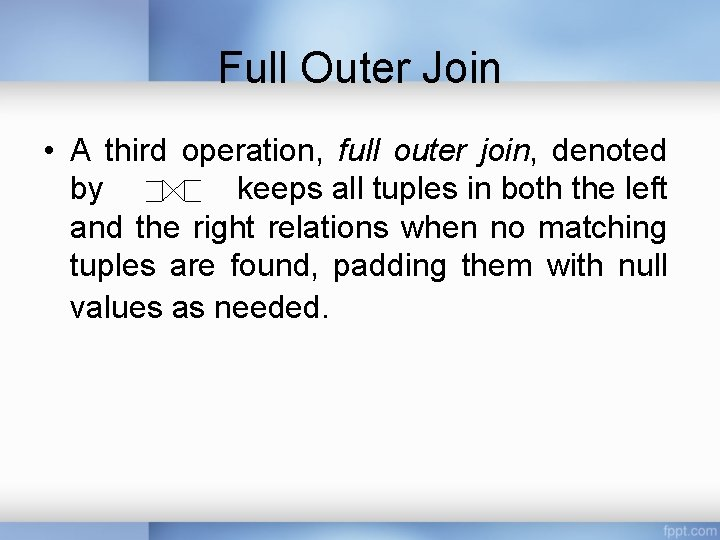Full Outer Join • A third operation, full outer join, denoted by keeps all tuples in both the left and the right relations when no matching tuples are found, padding them with null values as needed.ExampleLEFT OUTER JOIN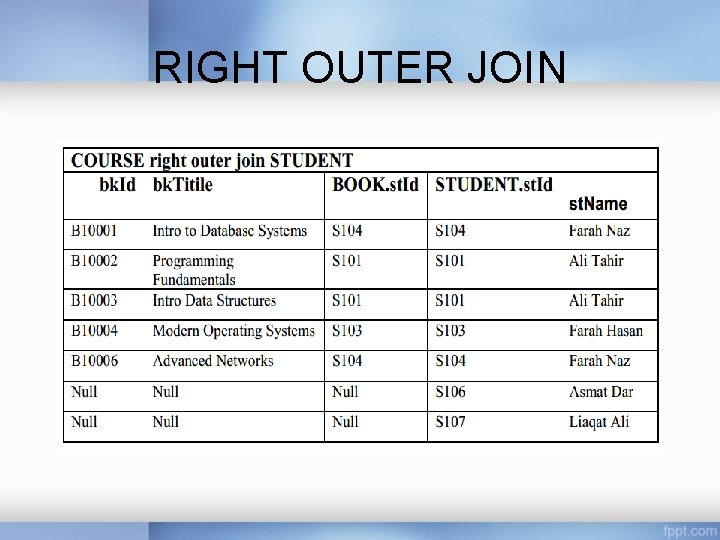RIGHT OUTER JOINFULL OUTER JOINSemi Join In semi join, first we take the natural join of two relations then we project the attributes of first table only. So after join and matching the common attribute of both relations only attributes of first relation are projected.Example 2Semi Join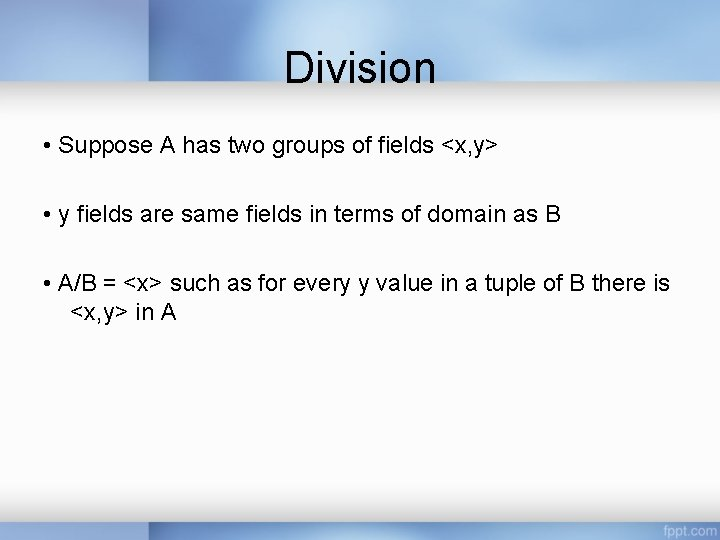Division • Suppose A has two groups of fields <x, y> • y fields are same fields in terms of domain as B • A/B = <x> such as for every y value in a tuple of B there is <x, y> in ADivisionDivision • Identify all clients who have viewed all properties with three rooms. ( client. No, property. No(Viewing)) ( property. No( rooms = 3 (Property. For. Rent)))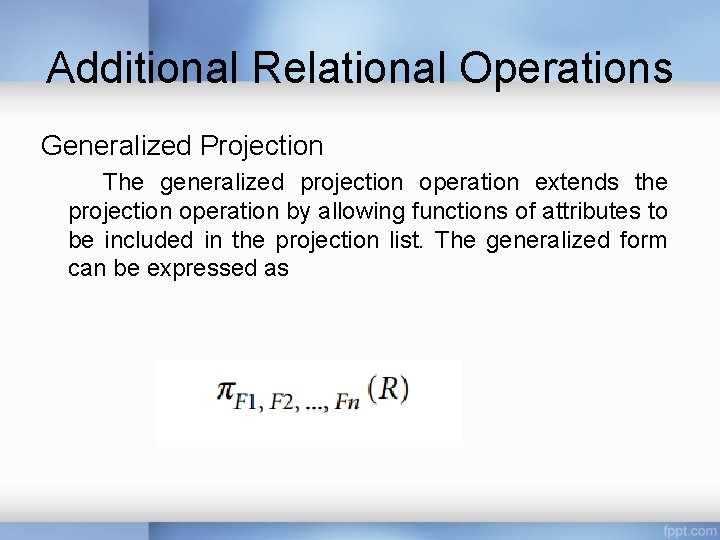Additional Relational Operations Generalized Projection The generalized projection operation extends the projection operation by allowing functions of attributes to be included in the projection list. The generalized form can be expressed asGeneralized ProjectionAggregate functions and grouping •  ommon functions applied to collections of numeric values • Include SUM, AVERAGE, MAXIMUM, and MINIMUMAggregate functions • Group tuples by the value of some of their attributes • Apply aggregate function independently to each group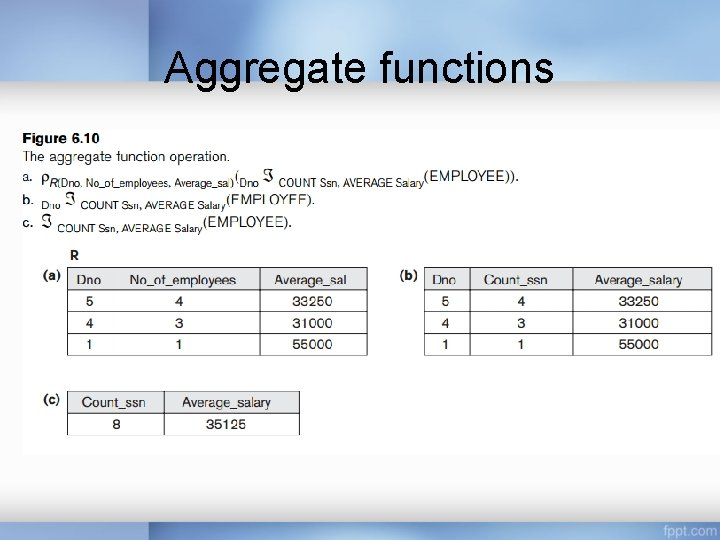Aggregate functions